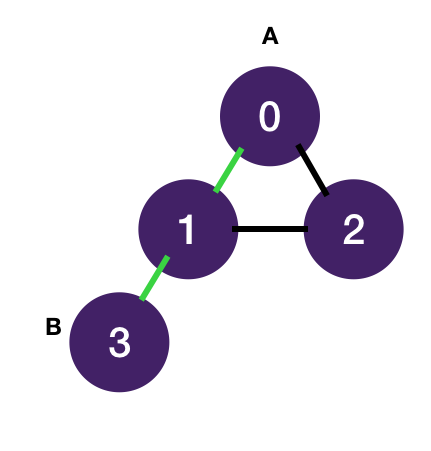# Shortest Path Between A and B

Prereq: BFS on Graph

Given an (unweighted) connected graph, return the length of the shortest path between two nodes A and B, in terms of the number of edges.

Input:

``````1graph = [[1, 2], [0, 2, 3], [0, 1], ]
2A = 0
3B = 3``````

Output: `2`Note that the `graph` is given as a 2-d list, where `graph[i]` is the list of nodes that node i is adjacent to. You can use the BFS template from BFS on graphs as your starter code.